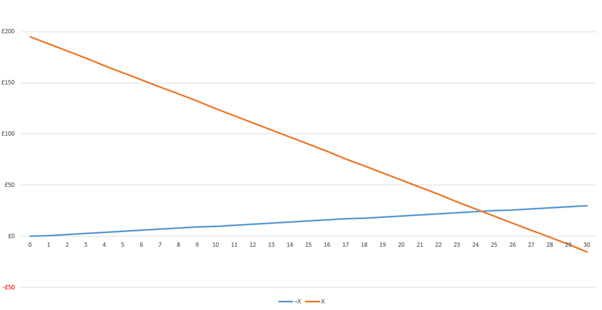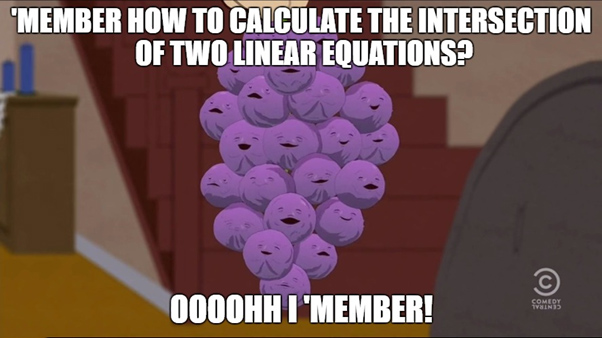# Optimisation of Matched Betting Choosing the Best Bet

Sat 5th Nov 2016

Free Money! There, that got you interested didn’t it! Well if you’ve come this far you probably already know what matched betting is and have a reasonable idea of how it works; you essentially try to liquidise the sign-up bonuses or the special offer free bets that bookies give you. Hence, free money! I'm not going to spend any time talking about the basics here, if you want to get into matched betting read up on it!

Now the maths behind the basics of matched betting is pretty simple. You bet your free bet at high odds with the bookie whilst simultaneously laying (betting against) the outcome of the same event at a betting exchange at odds that are as close to your bet odds as possible. The thing that I have however seen people take guess work with, is how much to actually place on the lay (and this makes me, in my world of perfect mathematical rules, very sad)! Usually people turn to online calculators, or they brute force the guess until the numbers of the winnings seem close enough, or even worse they just use the same amount for the lay as for the free bet.

By doing this, you’re actually introducing an element of risk [gamble] into your matched betting strategy as it is sub-optimal. Ideally you want to be living in a world where you’re placing the bet that whatever the outcome of the event, will give you the same (or as close to as) amount of payout as the inverse outcome.

How then, I hear you ask, do we work out the most optimal lay amount? Well, let’s start by first defining what we would call optimal, and let’s do this by using an example (everybody loves examples!):

Suppose you have a “free bet” of £30.
You’ve also found a good event that you think has good odds by your bookie and betting exchange. Your odds for outcome X on the event are 7.5 for backing and 8.0 for laying.

Backing the outcome X and that outcome subsequently happening nets you £195 (£30 * 7.5) - £30.
Backing the outcome X and the outcome not happening (¬X) gives you a loss of £0 (as you’ve just lost the free bet and not your own capital).
Whist at the same time Laying outcome ¬X will win you the amount you layed on (L) but if outcome X happens the lay will lose you -(8 * L) - L.

So the gross takings of outcome X are:
And the gross takings of outcome ¬X are:

To make all of the above optimal and reduce the risk [gamble] we need to get the gross winnings of either outcome be equal to each other, and hence we then truly won’t care about what the outcome is! And as you’ve probably noticed (mainly because I gave it away 3 paragraphs ago) to get those two amounts equal we need to optimise for L.

Now what we can notice about the series of L against the gross winnings of X and ¬X is that it varies linearly. If we were to plot lay amounts against the gross winnings we’d get a line! So let’s do that, let’s vary the lay amount (from £0-30) and plot the gross winnings as the Y amount for both outcomes X and ¬X:And look at that, we’ve got ourselves an intersecting line! And seen as the lines represent winnings for X and ¬X respectively we can say that those winnings are equal at the point where the lines intersect! As the x-axis is the lay amount, when we find out the x-coordinate of the point of intersection we’ll have found our optimal amount to lay!I know what you’re thinking right now “man I wish I’d paid more attention to that maths lesson when I was 12 years old rather than trying to flick chewing gum into that nerdy kid’s hair!”. Well luckily for you I was that nerdy kid (and my hair is finally all clean, thank you!) and I happen to remember how to calculate the intersection of two linear equations.

Simple; you make both equations equal to the y term and then make them equal to each other and solve for x. Remember that a linear equation is in the form of  (where c is the y-axis intercept and m is the gradient).

Now you’re wondering “okay, but how do we get the linear equations from the back and lay odds?” well if we remember back to that teen-maths lessons, the gradient of a line is worked out by taking the change in y divided by the change in x. So let’s kill two birds with one stone and get c (the intercept) at the same time as getting m (the gradient). The intercept is easy, just set x = 0 and we get c. For our example, this works out as:

For c and for the gradients m we get:

And hence our two linear equations for the two outcomes are:

Setting the equations equal to each other gives:

And finally solving for x (I’m not going to do a step by step for super-basic algebra here, I think the other nerdy kid who you locked in the toilet is free to give you revision lessons with that one):

Now let’s see what happens when we take that solved intersection x-value and plug it into our original final winnings (rounding for currency)!

Winner! We have now solved for a fully optimal bet! So laying an amount of £24.38 will guarantee you the most even, risk-free profit despite the outcome!

## Hold up! What about Minimising a Loss?

You see, a lot of these free bet offers you will come across will require you to bet some of your own money at certain odds or higher to unlock the free bet to your account! With these bets you’re risking your own capital on both sides of the bet and hence with a less than perfect margin in the bet/lay spreads you will make a loss. What you want to do is minimise the loss as optimally as you can.

Well, we can use exactly the same principle as above, the only change we would make is to factor in the –bet in the ¬X outcome loss. So as an example, we have a £10 bet we need to make at odds > 2.0. We found an event with odds 2.1 X and 2.12 ¬X. So using the equations above we make the 2 linear equations from those again. These work out at:

Solving for these in respect of x gives  and hence substituting that back into the original gross loss scenarios gives us a loss of £0.09 on either outcome. The optimal loss scenario!

Now, I know everybody's lazy and if you've made it down this far in the article (without cheating and just scrolling down!) I'll reward you with an Excel spreadsheet that uses the equations above to calculate your optimal Lay amount for both free bet maximisation and own capital minimisation based on the bet amount and specified odds. Download it Here